# Building a book Recommendation System using Keras

https://towardsdatascience.com/building-a-book-recommendation-system-using-keras-1fba34180699

## How to use embeddings to create a book recommendation system.Figure 1: Photo by Brandi Redd on Unsplash

An recommendation system seeks to predict the rating or preference a user would give to an item given his old item ratings or preferences. Recommendation systems are used by pretty much every major company in order to enhance the quality of there services.

In this article, we will take a look at how to use embeddings to create a book recommendation system.

For our data we will use the goodbooks-10k dataset which contains ten thousand different books and about one million ratings. It has three features the book_id, user_id and rating. If you don’t want to download the dataset from Kaggle yourself you can get the file as well as the complete code covered in this article from my Github repository.

### Embedding

An embedding is a mapping from discrete objects, such as words or ids of books in our case, to a vector of continuous values. This can be used to find similarities between the discrete objects, that wouldn’t be apparent to the model if it didn’t use embedding layers.

The embedding vectors are low-dimensional and get updated whilst training the network. The image below shows an example of embedding created using Tensorflows Embedding Projector.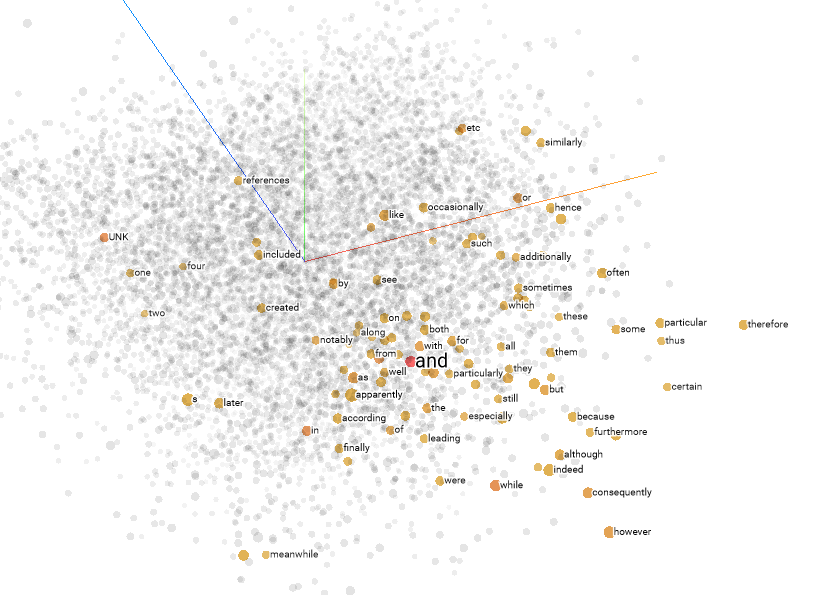Figure 2: Projector Embeddings

### Getting data

Pandas will be used for loading in the dataset. Then the data will be split into a training and testing set and we will create two variables that give us the unique number of users and books.

dataset = pd.read_csv('ratings.csv')
train, test = train_test_split(dataset, test_size=0.2, random_state=42)
n_users = len(dataset.user_id.unique())
n_books = len(dataset.book_id.unique())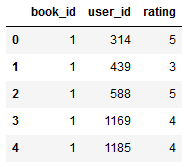Figure 3: Rating-Dataset Head

The dataset is already cleaned so we don’t need to take any further data cleaning or preprocessing steps.

### Creating Embedding Model

The Keras deep learning framework makes it easy to create neural network embeddings as well as working with multiple input and output layers.

Our model will have the following structure:

1. Input: Input for both books and users
2. Embedding Layers: Embeddings for books and users
3. Dot: combines embeddings using a dot product

In an embedding model the embeddings are the weights that are learned during training. These embeddings can not only be used for extracting information about the data but can also be extracted and visualized.

For simplicity reasons I didn’t add any fully-connected layers at the end, eventhough this could have increased the accuracy by quite a bit. So if you want a more accurate model this is something to try out.

Below is the code for creating the model:

from keras.layers import Input, Embedding, Flatten, Dot, Dense
from keras.models import Model
book_input = Input(shape=, name="Book-Input")
book_embedding = Embedding(n_books, 5, name="Book-Embedding")(book_input)
book_vec = Flatten(name="Flatten-Books")(book_embedding)
user_input = Input(shape=, name="User-Input")
user_embedding = Embedding(n_users, 5, name="User-Embedding")(user_input)
user_vec = Flatten(name="Flatten-Users")(user_embedding)
prod = Dot(name="Dot-Product", axes=1)([book_vec, user_vec])
model = Model([user_input, book_input], prod)
model.compile('adam', 'mean_squared_error')

### Training the model

Now that we have created our model we are ready to train it. Because we have two input layers (one for the books and one for the users) we need to specify an array of training data as our x data. For this article I trained the model for 10 epochs but you can train it longer if you want to get better results.

Below is the training code:

history = model.fit([train.user_id, train.book_id], train.rating, epochs=10, verbose=1)
model.save('regression_model.h5')

### Visualizing embeddings

Embeddings can be used to visualize concepts such as the relation of different books in our case. To visualize these concepts we need to further reduce dimensionality using dimensionality reduction techniques like principal component analysis (PSA) or t-distributed stochastic neighbor embedding (TSNE).

Starting with 10000 dimensions (on for each book), we map them to 5 dimensions using embedding and then further map them to 2 dimensions using PCA or TSNE.

First of we need to extract the embeddings using the get_layer function:

# Extract embeddings
book_em = model.get_layer('Book-Embedding')
book_em_weights = book_em.get_weights()

Now we will use PCA to transform our embeddings to 2 dimensions and then scatter the results using Seaborn:

from sklearn.decomposition import PCA
import seaborn as sns
pca = PCA(n_components=2)
pca_result = pca.fit_transform(book_em_weights)
sns.scatterplot(x=pca_result[:,0], y=pca_result[:,1])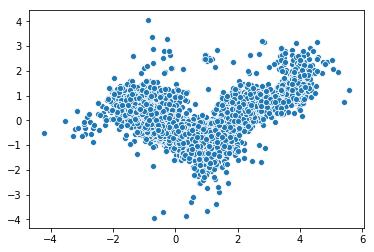Figure 4: Visualizing embeddings with PCA

The same can be done using TSNE:

from sklearn.manifold import TSNE
tsne = TSNE(n_components=2, verbose=1, perplexity=40, n_iter=300)
tnse_results = tsne.fit_transform(book_em_weights)
sns.scatterplot(x=tnse_results[:,0], y=tnse_results[:,1])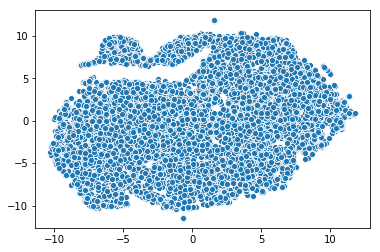Figure 5: Visualizing embeddings with TSNE

### Making Recommendations

Making recommendations using our trained model is simple. We only need to feed in a user and all books and then select the books which have the highest predicted ratings for that specific user.

The code below shows the process of making prediction for a specific user:

# Creating dataset for making recommendations for the first user
book_data = np.array(list(set(dataset.book_id)))
user = np.array([1 for i in range(len(book_data))])
predictions = model.predict([user, book_data])
predictions = np.array([a for a in predictions])
recommended_book_ids = (-predictions).argsort()[:5]
print(recommended_book_ids)
print(predictions[recommended_book_ids])

This code outputs:

array([4942, 7638, 8853, 9079, 9841], dtype=int64)
array([5.341809 , 5.159592 , 4.9970446, 4.9722786, 4.903894 ], dtype=float32)

We can use the book ids to get more information about the books using the books.csv file.

books = pd.read_csv(‘books.csv’)
books.head()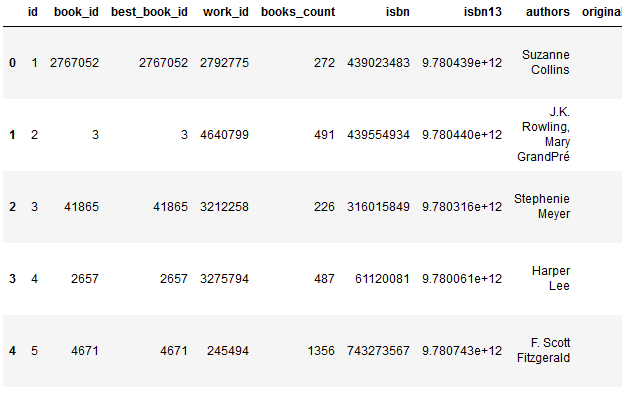Figure 6: Book-Dataset Head

print(books[books[‘id’].isin(recommended_book_ids)])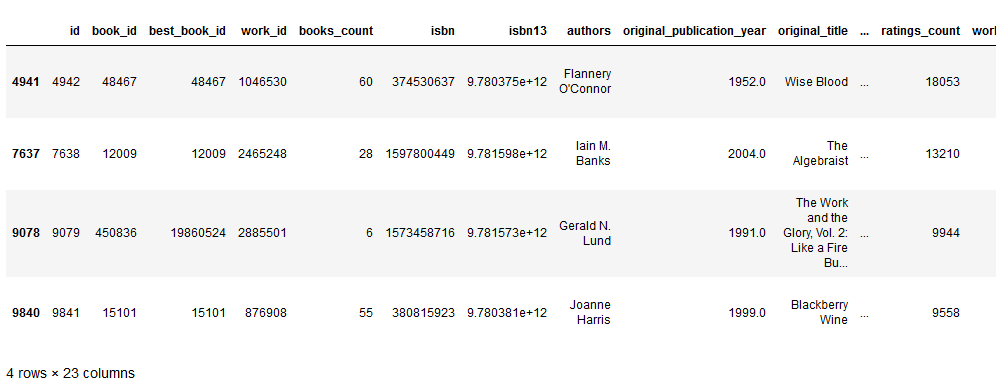Figure 7: Recommended books

### Conclusions

Embeddings are a method of mapping from discrete objects, such as words to vectors of continuous values. They are useful for finding similarities, visualization purposes and as an input into another machine learning model.

This example certainly isn’t perfect and there are a lot of things that can be tried in order to get better performance but it is a good starting point for learning how to use embeddings for more advanced problems.

A few things you could add to get better results:

• Add fully-connected layers after the dot product
• Train for more epochs
• Scale the ratings column
• etc.

If you liked this article consider subscribing on my Youtube Channel and following me on social media.

If you have any question or critic I can be reached via Twitter or the comment section.